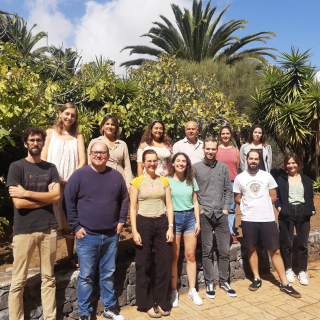# The SAMI Galaxy Survey: Stellar Population Gradients of Central Galaxies

Santucci, Giulia; Brough, Sarah; Scott, Nicholas; Montes, Mireia; Owers, Matt S.; van Sande, Jesse; Bland-Hawthorn, Joss; Bryant, Julia J.; Croom, Scott M.; Ferreras, Ignacio; Lawrence, Jon S.; López-Sánchez, Ángel R.; Richards, Samuel N.
Bibliographical reference

The Astrophysical Journal

6
2020
Number of authors
13
IAC number of authors
1
Citations
28
Refereed citations
28
Description
We examine the stellar population radial gradients (age, metallicity, and [α/Fe]) of 96 passive central galaxies up to ∼2 Re in the SAMI Galaxy Survey. The targeted groups have a halo mass range of $11\lt \mathrm{log}({M}_{200}/{M}_{\odot })\lt 15$ . The main goal of this work is to determine whether central galaxies have different stellar population properties when compared to similarly massive satellite galaxies. For the whole sample, we find negative metallicity radial gradients, which show evidence of becoming shallower with increasing stellar mass. The age and [α/Fe] gradients are slightly positive and consistent with zero, respectively. The [α/Fe] gradients become more negative with increasing mass, while the age gradients do not show any significant trend with mass. We do not observe a significant difference between the stellar population gradients of central and satellite galaxies at fixed stellar mass. The mean metallicity gradients are $\overline{{\rm{\Delta }}[{\rm{Z}}/{\rm{H}}]/{\rm{\Delta }}\mathrm{log}(r/{R}_{e})}=-0.25\pm 0.03$ for central galaxies and $\overline{{\rm{\Delta }}[{\rm{Z}}/{\rm{H}}]/{\rm{\Delta }}\mathrm{log}(r/{R}_{e})}=-0.30\pm 0.01$ for satellites. The mean age and [α/Fe] gradients are consistent between central and satellite galaxies, within the uncertainties, with a mean value of $\overline{{\rm{\Delta }}{\rm{log(Age/Gyr)}}/{\rm{\Delta }}\mathrm{log}(r/{R}_{e})}=0.13\pm 0.03$ for centrals and $\overline{{\rm{\Delta }}{\rm{log(Age/Gyr)}}/{\rm{\Delta }}\mathrm{log}(r/{R}_{e})}=0.17\pm 0.01$ for satellites and $\overline{{\rm{\Delta }}[\alpha /\mathrm{Fe}]/{\rm{\Delta }}\mathrm{log}(r/{R}_{e})}=0.01\pm 0.03$ for centrals and $\overline{{\rm{\Delta }}[\alpha /\mathrm{Fe}]/{\rm{\Delta }}\mathrm{log}(r/{R}_{e})}=0.08\pm 0.01$ for satellites. The stellar population gradients of central and satellite galaxies show no difference as a function of halo mass. This evidence suggests that the inner regions of central passive galaxies form in a similar fashion to those of satellite passive galaxies, in agreement with a two-phase formation scenario.
Related projectsTraces of Galaxy Formation: Stellar populations, Dynamics and Morphology

We are a large, diverse, and very active research group aiming to provide a comprehensive picture for the formation of galaxies in the Universe. Rooted in detailed stellar population analysis, we are constantly exploring and developing new tools and ideas to understand how galaxies came to be what we now observe.

Ignacio
Martín Navarro
Type